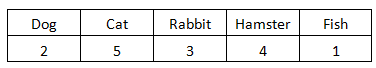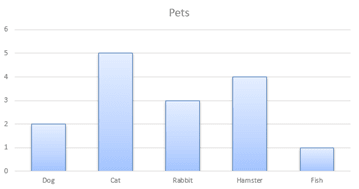The K5 Learning Blog urges parents to be pro-active in helping their children reach their full academic potential.

K5 Learning
provides free worksheets, workbooks and an online reading and math program for kindergarten to grade 5 students.# How to find the Mean of a Bar Graph

In a previous post we showed you how to work out the mode of a bar chart, also called a bar graph. This time, we’ll look at how to work out the mean of a bar chart.

Let’s use the same example. A group of students were surveyed on what pets their families had. Here are the results:

##Survey results show in bar graph

Show in a bar graph, the results look like this:## What is the mean of a bar graph?

The mean is also called the average. To find the mean, we’ll need to:

The numbers in this example are 2, 5, 3, 4, 1

2.    Divide the sum by the number of addends.

The number of addends in this example are 5.

Here’s how to work out the mean of our example:

2 + 5 + 3 + 4 + 1 = 15

15 / 5 = 3

The mean number of pets is 3.